Courses

# Past Year Questions: Properties of Soils Notes | EduRev

## Civil Engineering (CE) : Past Year Questions: Properties of Soils Notes | EduRev

The document Past Year Questions: Properties of Soils Notes | EduRev is a part of the Civil Engineering (CE) Course Topic wise GATE Past Year Papers for Civil Engineering.
All you need of Civil Engineering (CE) at this link: Civil Engineering (CE)

Q.1 The notation "SC" as per Indian Standard Soil Classification System refers to
(a) Silty clay
(b) Clayey sand
(c) Sandv clav
(d) Clavev silt
Ans.
(B)
Solution:
SC → Clayey sand

Q.2  A soil has specific gravity of its solids equal to 2.65. The mass density of water is 1000 kg/m3. Considering zero air voids and 10% moisture content of the soil sample, the dry density (in kg/m3, round off to 1 decimal place) would be _______.    [2019 : 1 Mark, Set-I]
Solution:

w - 10% and has zero air voids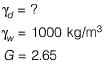Zero air voids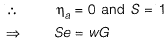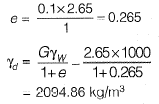Q.3  The clay mineral, whose structural units are held together by potassium bond is    [2018 : 1 Mark, Set-II]
(a) Halloysite
(b) lilite
(c) Kaolinite
(d) Smectite
Ans.
(B)
Solution:
Kaolinite → Hydrogen bond
lllite → Ionic Bond/Potassium Bond
Montmorillonite → Water Bond

Q.4  in a shrinkage limit test, the volume and mass of a dry soil pat are found to be 50 cm3 and 88 g. respectively. The specific gravity of the soil solids is 2.71 and the density of water is 1 g/cc. The shrinkage limit (in % up to two decimal places) is ________ .    [2018 : 1 Mark, Set-I]
Solution:

Dry soil mass = 88 gm
Volume of dry soil = 50 cc
Dry density of soil mass,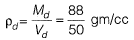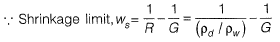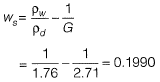Q.5 Let G be the specific gravity of soil solids, w is the water content in the soil sample, γthe unit weight of water, and γd the dry unit weight of the soil. The equation for the zero air voids line in a compaction test plot is    [2017 : 1 Mark, Set-II]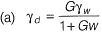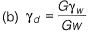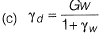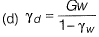Ans.
(A)
Solution:
Percentage air void line is relation between dry unit weight and water content at constant air void. Hence equation of zero air void line is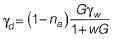(na = 0)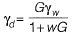Q.6 The laboratory tests on a soil sample yield the following results; natural moisture content = 18%, liquid limit = 60%, plastic limit = 25%, percentage of clay sized fraction = 25%. The liquidity index and activity (as per the expression proposed by Skempton) of the soil, respectively, are    [2017 : 2 Marks, Set-I]
(a) -0.2 and 1,4
(b) 0.2 and 1.4
(c) -1.2 and 0.714
(d) 1.2 and 0.714
Ans.
(A)
Solution:
Given data: wn = 18%, wL = 60%, wp = 25% % of clay size particle = 25%
Liquidity index,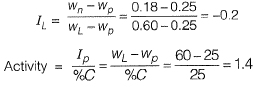Q.7 The porosity (n) and the degree of saturation (S) of a soil sample are 0.7 and 40%, respectively. In a 100 m3 volume of the soil, the volume (expressed in m3) of air is _____    [2016 : 2 Marks, Set-I]
Solution: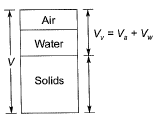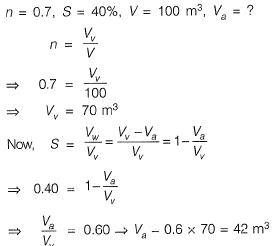Q.8 A 588 cm3 volume of moist sand weighs 1010 gm. its dry weight is 918 gm and specific gravity of solids, G is 2.67. Assuming density of water as 1 gm/cm3, the void ratio is _________ .     [2015 : 2 Marks, Set-II]
Solution: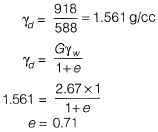Q.9 If the water content of a fully saturated soil mass is 100%, the void ratio of the sample is
(a) less than specific gravity of soil
(b) equal to specific gravity of soil
(c) greater than specific gravity of soil
(d) independent of specific gravity of soil
Ans.
(B)
Solution: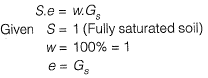Q.10 An earth embankment is to be constructed with compacted cohesionless soil. The volume of the embankment is 5000 m3 and the target dry unit weight is 16.2 kN/m3. Three nearby sites (see figure below) have been identified from where the required soil can be transported to the construction site. The void ratios (e) of different sites are shown in the figure. Assume the specific gravity of soil to be 2.7 for ail three sites. If the cost of transportation per km is twice the cost of excavation per m3 of borrow pits, which site would you choose as the most economic solution? (Use unit weight of water = 10 kN/m3)    [2015 : 2 Marks, Set-I]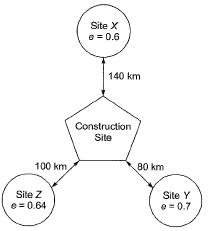(a) Site X
(b) Site Y
(c) Site Z
(d) Any of the sites
Ans.
(A)
Solution:
Volume of solids at embankment,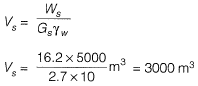Volume of solids will remain constant,
also,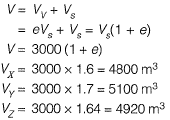Let cost of excavation be α/m3,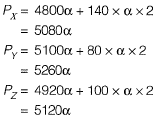Hence Px is least.
∴ Hence total cost for site X is least.

Q.11 A fine-grained soil has 60% (by weight) silt content. The soil behaves as semi-solid when water content is between 15% and 28%. The soil behaves fluidlike when the water content is more than 40%.
The ‘Activity’ of the soil is    [2015 : 1 Mark, Set-I]
(a) 3.33
(b) 0.42
(c) 0.30
(d) 0.20
Ans.
(C)
Solution: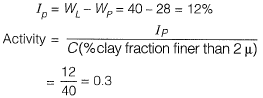Q.12 A certain soil has the following properties: Gs = 2.71, n - 40% and w= 20%. The degree of saturation of the soil (rounded off to the nearest percent) is _________ .    [2014 : 1 Mark, Set-II]
Solution:

Se = wG
and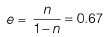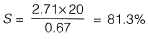Q.13  A given cohesionless soil has emax = 0.85 and emin = 0.50. In the field, the soil is compacted to a mass density of 1800 kg/m3 at a water content of 8%. Take the mass density of water as 1000 kg/m3 and Gs as 2.7. The relative density (in %) of the soil is    [2014 : 2 Marks, Set-I]
(a) 56.43
(b) 60.25
(c) 62.87
(d) 65.71
Ans.
(D)
Solution: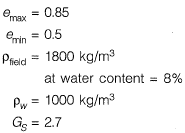Relative density, ID = ?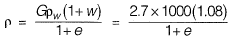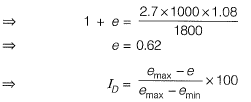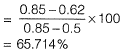Q.14 In its natural condition a soil sample has a mass of 1.980 kg and a volume of 0.001 m3. After being completely dried in an oven; the mass of the sample is 1.800 kg. Specific gravity is 2.7. Unit weight of water is 10 kN/m3. The degree of saturation of soil is    [2013 : 1 Mark]
(a) 0.65
(b) 0.7
(c) 0.54
(d) 0.61
Ans.
(C)
Solution: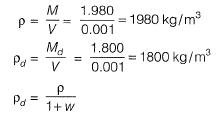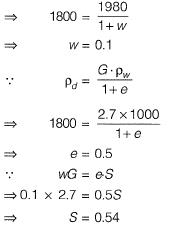Q.15 A soil is composed of solid spherical grains of identical specific gravity and diameter between 0.075 mm and 0.0075 mm. If the terminal velocity of the largest particle falling through water without flocculation is 0.5 mm/s, that for the smallest particle would be    [2011 : 1 Mark]
(a) 0.005 mm/s
(b) 0.05 mm/s
(c) 5 mm/s
(d) 50 mm/s
Ans.
(A)
Solution:
According to Stake’s law,
V ∝ D2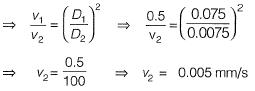Offer running on EduRev: Apply code STAYHOME200 to get INR 200 off on our premium plan EduRev Infinity!

67 docs

,

,

,

,

,

,

,

,

,

,

,

,

,

,

,

,

,

,

,

,

,

;